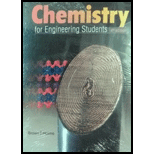# 3.60 Copper can have improved wear resistance if alloyed with ceramic alumina, A1 2 O 3 . If a copper alloy has 8.5 wt% A1 2 O 3 , what is its composition in mol%?### Chemistry for Engineering Students

4th Edition
Lawrence S. Brown + 1 other
Publisher: Cengage Learning
ISBN: 9781337398909### Chemistry for Engineering Students

4th Edition
Lawrence S. Brown + 1 other
Publisher: Cengage Learning
ISBN: 9781337398909
Chapter 3, Problem 3.60PAE
Textbook Problem
6 views

## 3.60 Copper can have improved wear resistance if alloyed with ceramic alumina, A12O3. If a copper alloy has 8.5 wt% A12O3,what is its composition in mol%?

Interpretation Introduction

Interpretation:

Mol % of the elements present in alloy are calculated

Concept Introduction:

Mol % are calculated using the formula

% moles of element = molesofelementtotalmoles×100

To determine:

Copper alloy has 8.5 wt% Al2O3, what is its composition in mol%?

### Explanation of Solution

Assume the total weight of the alloy is 100 g

Wt % of Al2O3 = 8.5 % which means that Al2O3 is 8.5 g in the 100 g of the sample

Weight of copper = 100-8.5= 91.5 g

Molar mass of Cu= 63.05 g/mol

Molar mass of Al2O3 = 102 g/mol

Moles = givenmass(g)molarmass

Moles of Cu= 91.5g63.5g/mol=1

### Still sussing out bartleby?

Check out a sample textbook solution.

See a sample solution

#### The Solution to Your Study Problems

Bartleby provides explanations to thousands of textbook problems written by our experts, many with advanced degrees!

Get Started

Find more solutions based on key concepts
What differentiates innate immunity from adaptive (acquired, immunity?

Introduction to General, Organic and Biochemistry

Fasting hypoglycemia maybe caused by all except a. pancreatic tumors. b. poorly controlled diabetes. c. overuse...

Nutrition: Concepts and Controversies - Standalone book (MindTap Course List)

Why are interstellar lines so narrow?

Horizons: Exploring the Universe (MindTap Course List)

A standard unit must have what characteristics?

An Introduction to Physical Science

A ball having mass m is fastened at the end of a flagpole that is connected to the side of a tall building at p...

Physics for Scientists and Engineers, Technology Update (No access codes included)

Plankton are said to be drifters, yet some of them can swim. Which is it?

Oceanography: An Invitation To Marine Science, Loose-leaf Versin